# PSAT Math : Geometry

## Example Questions

### Example Question #6 : Rectangles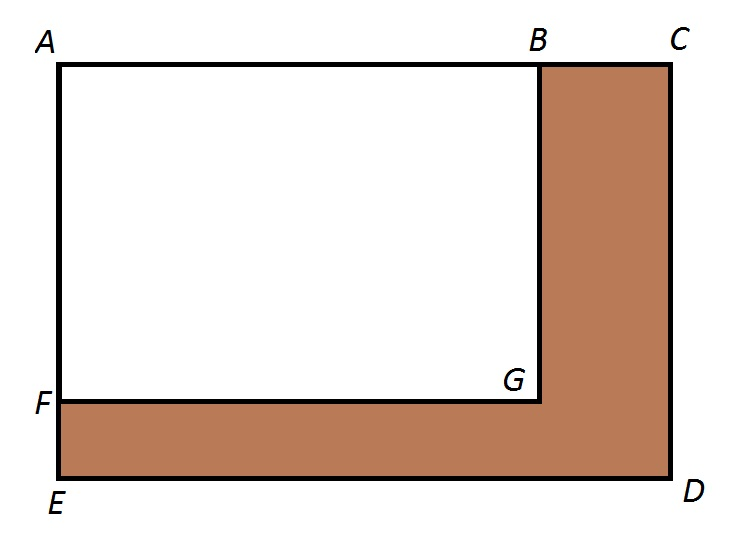Note: Figure NOT drawn to scale.

Refer to the above figure.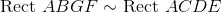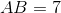and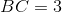.

What percent of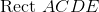has been shaded brown ?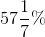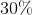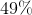Insufficient information is given to answer the problem.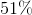Explanation:and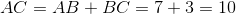, so the similarity ratio ofto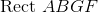is 10 to 7. The ratio of the areas is the square of this, or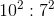or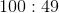Therefore,comprises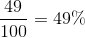of, and the remainder of the rectangle - the brown region - is 51% of.

### Example Question #7 : Rectangles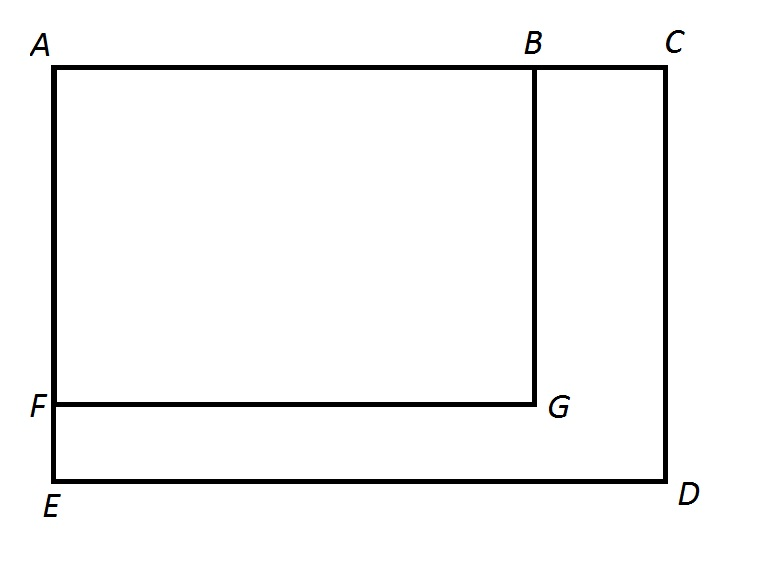Note: figure NOT drawn to scale.

Refer to the above figure.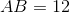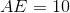.

Give the area of.

.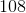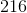Explanation:, so the sides are in proportion - that is,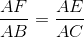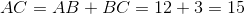Set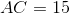and solve for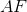: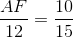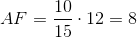has area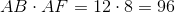### Example Question #1911 : Hspt Mathematics

A rectangle has a width of 2x. If the length is five more than 150% of the width, what is the perimeter of the rectangle?

6x2 + 5

5x + 5

6x2 + 10x

5x + 10

10(x + 1)

10(x + 1)

Explanation:

Given that w = 2x and l = 1.5w + 5, a substitution will show that l = 1.5(2x) + 5 = 3x + 5.

P = 2w + 2l = 2(2x) + 2(3x + 5) = 4x + 6x + 10 = 10x + 10 = 10(x + 1)

### Example Question #11 : Rectangles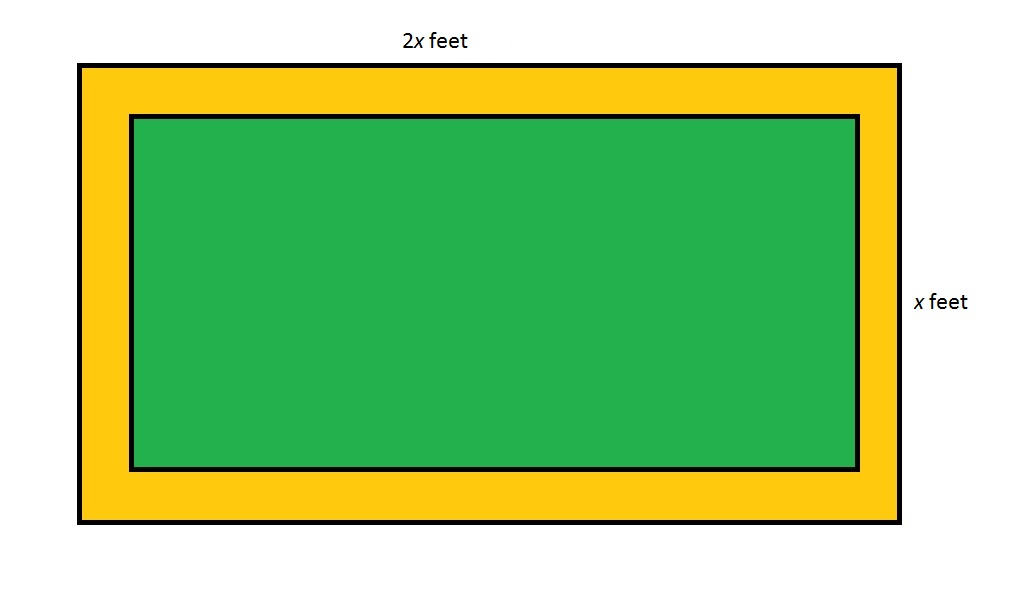Note: Figure NOT drawn to scale

Refer to the above figure, which shows a rectangular garden (in green) surrounded by a dirt path (in orange). The dirt path is seven feet wide throughout. Which of the following polynomials gives the perimeter of the garden in feet?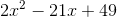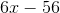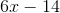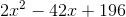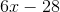Explanation:

The length of the garden, in feet, is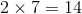feet less than that of the entire lot, or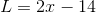;

The width of the garden, in feet, isless than that of the entire lot, or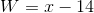.

The perimeter, in feet, is twice the sum of the two: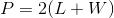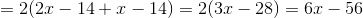### Example Question #12 : Rectangles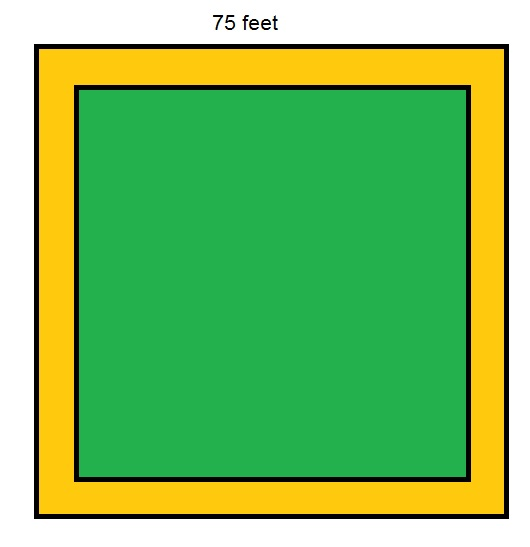Note:  Figure NOT drawn to scale

Refer to the above figure, which shows a square garden (in green) surrounded by a dirt path (in orange) eight feet wide throughout. What is the perimeter of the garden?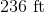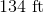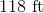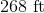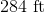Explanation:

The inner square, which represents the garden, has sidelength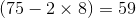feet, so its perimeter is four times this: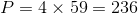feet.

### Example Question #631 : Geometry

Farmer Dave has a rectangular field that is 50 yards wide and 40 yards long. He wants to enclose the field with a wire fence. How much wire does Farmer Dave need?

170 yards

210 yards

160 yards

180 yards

200 yards

180 yards

Explanation:

To solve this problem, find the perimeter of the rectangle. There are two sides that each measure 50 yards and two sides that each measure 40 yards. Together these four sides measure 180 yards.

### Example Question #632 : Geometry

A rectangular garden has an area of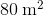. Its length ismeters longer than its width. How much fencing is needed to enclose the garden?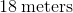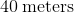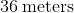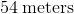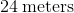Explanation:

We define the variables as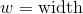and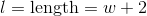.

We substitute these values into the equation for the area of a rectangle and get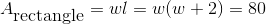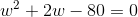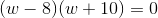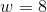or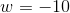Lengths cannot be negative, so the only correct answer is. If, then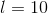Therefore,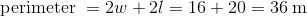.

### Example Question #7 : Quadrilaterals

A contractor is going to re-tile a rectangular section of the kitchen floor. If the floor is 6ft x 3ft, and he is going to use square tiles with a side of 9in. How many tiles will be needed?

24

40

32

2

32

Explanation:

We have to be careful of our units. The floor is given in feet and the tile in inches. Since the floor is 6ft x 3ft. we can say it is 72in x 36in, because 12 inches equals 1 foot. If the tiles are 9in x 9in we can fit 8 tiles along the length and 4 tiles along the width. To find the total number of tiles we multiply 8 x 4 = 32. Alternately we could find the area of the floor (72 x 36, and divide by the area of the tile 9 x 9)

### Example Question #8 : Quadrilaterals

The rectangular bathroom floor in Michael’s house is ten feet by twelve feet. He wants to purchase square tiles that are four inches long and four inches wide to cover the bathroom floor. If each square tile costs $2.50, how much money will Michael need to spend in order to purchase enough tiles to cover his entire bathroom floor? Possible Answers:$5400

$4800$1080

$1920$2700

$2700 Explanation: The dimensions for the bathroom are given in feet, but the dimensions of the tiles are given in inches; therefore, we need to convert the dimensions of the bathroom from feet to inches, because we can’t compare measurements easily unless we are using the same type of units. Because there are twelve inches in a foot, we need to multiply the number of feet by twelve to convert from feet to inches. 10 feet = 10 x 12 inches = 120 inches 12 feet = 12 x 12 inches = 144 inches This means that the bathroom floor is 120 inches by 144 inches. The area of Michael’s bathroom is therefore 120 x 144 in2 = 17280 in2. Now, we need to find the area of the tiles in square inches and calculate how many tiles it would take to cover 17280 in2. Each tile is 4 in by 4 in, so the area of each tile is 4 x 4 in2, or 16 in2. If there are 17280 in2 to be covered, and each tile is 16 in2, then the number of tiles we need is 17280 ÷ 16, which is 1080 tiles. The question ultimately asks us for the cost of all these tiles; therefore, we need to multiply 1080 by 2.50, which is the price of each tile. The total cost = 1080 x 2.50 dollars = 2700 dollars. The answer is$2700.

### Example Question #9 : Quadrilaterals

Ron has a fixed length of wire that he uses to make a lot. On Monday, he uses the wire to make a rectangular lot. On Tuesday, he uses the same length of wire to form a square-shaped lot. Ron notices that the square lot has slightly more area, and he determines that the difference between the areas of the two lots is sixteen square units. What is the positive difference, in units, between the length and the width of the lot on Monday?

6

10

4

12

8

8

Explanation:

Let’s say that the rectangular lot on Monday has a length of l and a width of w. The area of a rectangular is the product of the length and the width, so we can write the area of the lot on Monday as lw.

Next, we need to find an expression for the area of the lot on Tuesday. We are told that the lot is in the shape of a square and that it uses the same length of wire. If the length of the wire used is the same on both days, then the perimeter will have to remain the same. In other words, the perimeter of the square will equal the perimeter of the rectangle. The perimeter of a rectangle is given by 2l + 2w.

We also know that if s is the length of a side of a square, then the perimeter is 4s, because each side of the square is congruent. Let’s write an equation that sets the perimeter of the rectangle and the square equal.

2l + 2w = 4s

If we divide both sides by 4 and then simplify the expression, then we can write the length of the square as follows: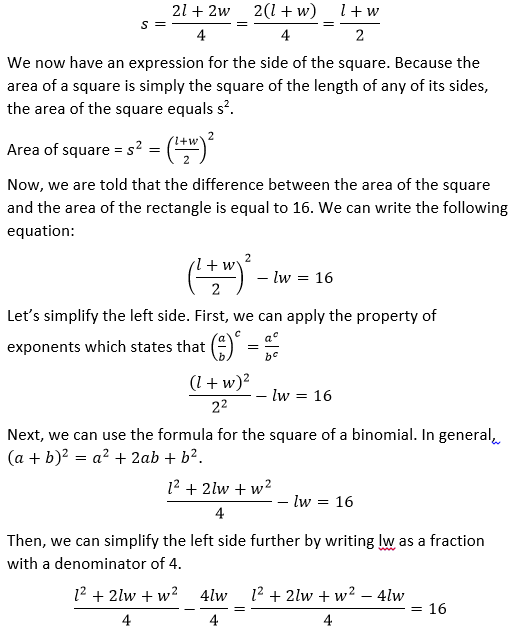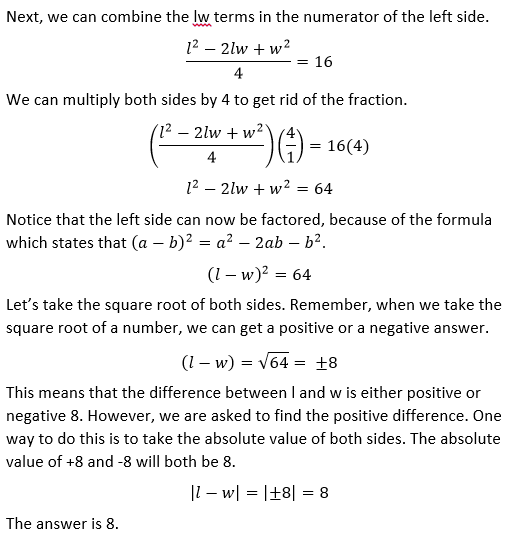### All PSAT Math Resources